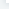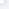# R语言中使用自定义函数

：2018-01-29 11:45:10

R语言中提供了大量的内置函数为用户解决有关问题，同时，R语言中允许用户编写自己的函数来解决实际问题。

R语言中定义自己的函数语法格式：

functionname <- function( arglist ) expr
return (value)

（1）无参函数

welcom<-function()
{
print("Welcome to my site:www.k88.net")
}

（2）带参数的函数

maxvalue<-function(x)
{
if(length(x)==0)
stop("参数x不能为空值。");
maxv<-x
for(i in 2:length(x))
if(x[i] > maxv)
maxv <- x[i]
return(maxv)
}

X<-c(11,34,21,38,71,29)
maxvalue(X)

（3）带默认参数的函数

maxnum<-function(n1,n2=0,n3)
{
maxn <- n1
if(n2 > maxn)
maxn <- n2
if(n3 > maxn)
maxn <- n3
return(maxn)
}

maxnum(1,2,3)
maxnum(1,n3=3)

R语言中使用自定义函数

## 相关文章